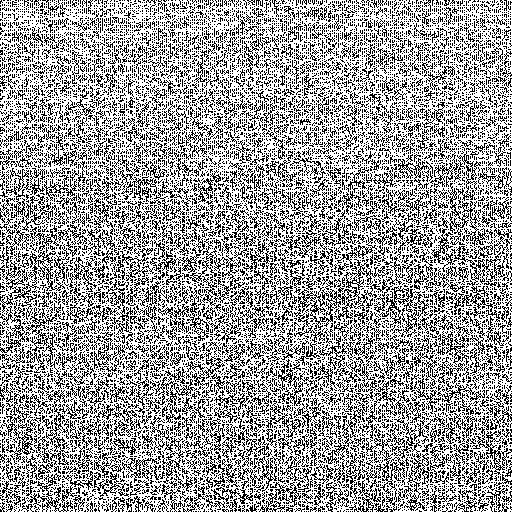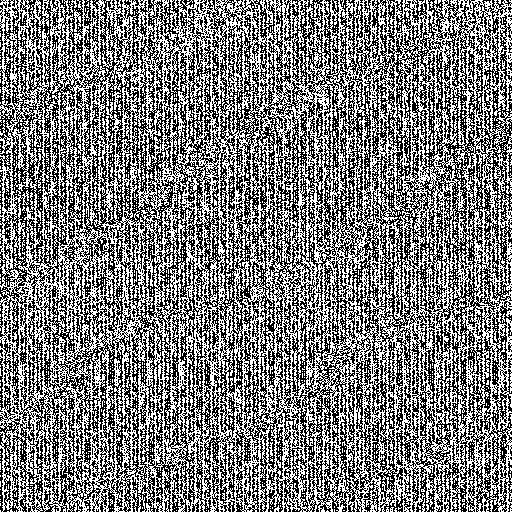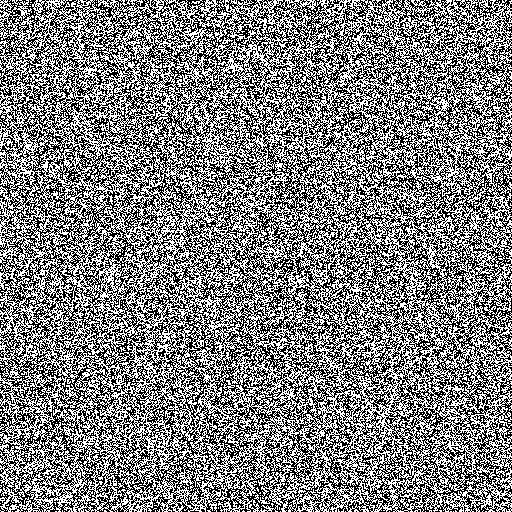# Poorman's Quite Random Number Generator (PQRNG) by Hardware

## Historycal document

The entropy source using ADC is same. The major difference is, NeuG uses CRC16 buffer to whiten the entropy source, and uses TinyMT parallel to CRC16 buffer output.

## Pretty good RNG

I think that I did make (or am making) pretty good hardware Random Numbere Generator.

It is a kind of True Random Number Generator (TRNG), but I am not sure how it is "true".

In 90's, I struggled "TrueIDE" mode of compact flash when I was developing GNU/Linux for SuperH. So, I don't want to say "True" when I am not absolutely sure.

It is not Pseudo RNG, as it is based on good entropy source. Thus, I call it P-Q-R NG, or "Poorman's Quite Random" number generator. "T" is somewhere over "S" after "P", "Q" and "R", and I don't know how far it is from here.

## Background

I want True Random Number Generator (TRNG). But, I can't find good one for my purpose (June 2011).

I investigate existing technology and understand that Zener diode with avalanche effect can be good entropy source, while meta-stability, PLL phase shift, or some external noise would be also candidates.

If I could design/build hardware, I'd adopt a circuit with Zener diode. Note that we need higher voltage for avalanche effect (more than 9V). Some people use voltage output of level convertor of RS232C (at least +9V/-9V).

## EntropyKey

While I investigate, I found EntropyKey, it says: it's a small, cheap, and easy device that provides your computers and servers with extra performance, security, and reliability.

## STM32F103

It seems that EntropyKey uses STM32F103, which is my favorite, too.

Yes, I am using STM32F103 for PQRNG. You can get an evaluation board of STM32F103 as cheap as 20 USD or so. If you have DIY skill and a JTAG debugger, you can use STM32 part of STM8S Discovery Kit by 750 JPY, that's pretty cheap.

Note that expensive version, STM32F2xx has built-in TRNG. If you don't have constraints like me, using STM32F2xx would be better choice.

## Entropy Sources

It is always problem to get some entropy for embedded device. I remember that I got difficulty to get randomness for my tiny TCP/IP implementation for M32R (in 2005).

Luckily, STM32F103 has built-in temperature sensor and voltage reference which connect to analog digital convertor (ADC).

For entropy source, we can use these signal outputs of temperature sensor and voltage reference, together with system tick of interrupt.

I did an experiment with entropy source:

```nib = (last two bits of TS) concatinated to (last two bits of VREF)
XOR (system tick >> 1)
```

Where TS means the value of temperature sensor measured by ADC and VREF means the value of voltage reference.

As I observed that LSB of system tick (SysTick->VAL in an expression of ChibiOS/RT) doesn't have good entropy, I don't use it.

Measured by MUST (L=8), I observed like 5.5-bit/byte entropy.

Next, I intentionally violate use of ADC. When we want correct values from ADC, we need to care about sample rate. Manual says that it should be 239.5 cycles. But, I use 1.5 cycles sample time. I don't know the exact reason, but I observed 6.0-bit/byte entropy with this setting.

## Whitening

I could say "true" if I were using entropy source as output directly. But, while the entropy source is good enough, it's not perfect for random number generation.

We need a filter or something which "whiten"s our entropy source.

I would say "quite", when I collect three words of 5.5-6.0bit/byte entropy source and output two words of random numbers, since "5.5 x 3 / 2" is more than 8.

I investigated the implementation of /dev/random of Linux, and studied how entropy source and entropy pool are managed. IIUC, entropy source are collected/filtered by a kind of TLFSR (twisted linear feedback shift register).

Entropy pool implementation like Linux's /dev/random is too much for me. I think that I don't need so many internal states, I just need simple one, such that three entropy inputs and two random number generations.

Looking the manual of STM32F103, I found that it has built-in CRC32 calculation unit. We can take advantage of this, I thought.

## Mersenne Twister and TinyMT

Looking a history of /dev/random implementation, I found that initial version was normal LFSR and "twisted" feature was added later. Then, I thought that it could be better to use MT (Mersenne Twister) instead of TLFSR to be modern.

Then, I happened to see annoucement of TinyMT. It's internal state is just four words.

"This is just for me", I thought. I decided to use TinyMT (instead of CRC32) to whiten the entropy source.

## Random byte images

We can see the difference of randomness by our eyes.

This is the one of randomness with normal ADC measurement. We see, this is somewhat light (5.5).This one is darker, the one of randomness with violating ADC measurement (6.0).This one is full randomness after the whitening filter.## Current experimental Code

I use Gnuk as a base of this experiment, since PQRNG will be used in Gnuk as well.

It is not integrated to Gnuk yet.

Here is current patch against Gnuk 0.13:

```diff --git a/ChibiOS_2.0.8/os/hal/platforms/STM32/adc_lld.h b/ChibiOS_2.0.8/os/hal/platforms/STM32/adc_lld.h
index cf2d4d4..05be6be 100644
@@ -238,26 +238,51 @@ typedef struct {
/* Driver macros.                                                            */
/*===========================================================================*/

+/**
+ * @brief   Number of channels in a conversion sequence.
+ */
#define ADC_SQR1_NUM_CH(n)      (((n) - 1) << 20)

-
-
+#define ADC_SQR3_SQ1_N(n)       ((n) << 0)  /**< @brief 1st channel in seq. */
+#define ADC_SQR3_SQ2_N(n)       ((n) << 5)  /**< @brief 2nd channel in seq. */
+#define ADC_SQR3_SQ3_N(n)       ((n) << 10) /**< @brief 3rd channel in seq. */
+#define ADC_SQR3_SQ4_N(n)       ((n) << 15) /**< @brief 4th channel in seq. */
+#define ADC_SQR3_SQ5_N(n)       ((n) << 20) /**< @brief 5th channel in seq. */
+#define ADC_SQR3_SQ6_N(n)       ((n) << 25) /**< @brief 6th channel in seq. */
+
+#define ADC_SQR2_SQ7_N(n)       ((n) << 0)  /**< @brief 7th channel in seq. */
+#define ADC_SQR2_SQ8_N(n)       ((n) << 5)  /**< @brief 8th channel in seq. */
+#define ADC_SQR2_SQ9_N(n)       ((n) << 10) /**< @brief 9th channel in seq. */
+#define ADC_SQR2_SQ10_N(n)      ((n) << 15) /**< @brief 10th channel in seq.*/
+#define ADC_SQR2_SQ11_N(n)      ((n) << 20) /**< @brief 11th channel in seq.*/
+#define ADC_SQR2_SQ12_N(n)      ((n) << 25) /**< @brief 12th channel in seq.*/
+
+#define ADC_SQR1_SQ13_N(n)      ((n) << 0)  /**< @brief 13th channel in seq.*/
+#define ADC_SQR1_SQ14_N(n)      ((n) << 5)  /**< @brief 14th channel in seq.*/
+#define ADC_SQR1_SQ15_N(n)      ((n) << 10) /**< @brief 15th channel in seq.*/
+#define ADC_SQR1_SQ16_N(n)      ((n) << 15) /**< @brief 16th channel in seq.*/
+
+#define ADC_SMPR2_SMP_AN0(n)    ((n) << 0)  /**< @brief AN0 sampling time.  */
+#define ADC_SMPR2_SMP_AN1(n)    ((n) << 3)  /**< @brief AN1 sampling time.  */
+#define ADC_SMPR2_SMP_AN2(n)    ((n) << 6)  /**< @brief AN2 sampling time.  */
+#define ADC_SMPR2_SMP_AN3(n)    ((n) << 9)  /**< @brief AN3 sampling time.  */
+#define ADC_SMPR2_SMP_AN4(n)    ((n) << 12) /**< @brief AN4 sampling time.  */
+#define ADC_SMPR2_SMP_AN5(n)    ((n) << 15) /**< @brief AN5 sampling time.  */
+#define ADC_SMPR2_SMP_AN6(n)    ((n) << 18) /**< @brief AN6 sampling time.  */
+#define ADC_SMPR2_SMP_AN7(n)    ((n) << 21) /**< @brief AN7 sampling time.  */
+#define ADC_SMPR2_SMP_AN8(n)    ((n) << 24) /**< @brief AN8 sampling time.  */
+#define ADC_SMPR2_SMP_AN9(n)    ((n) << 27) /**< @brief AN9 sampling time.  */
+
+#define ADC_SMPR1_SMP_AN10(n)   ((n) << 0)  /**< @brief AN10 sampling time. */
+#define ADC_SMPR1_SMP_AN11(n)   ((n) << 3)  /**< @brief AN11 sampling time. */
+#define ADC_SMPR1_SMP_AN12(n)   ((n) << 6)  /**< @brief AN12 sampling time. */
+#define ADC_SMPR1_SMP_AN13(n)   ((n) << 9)  /**< @brief AN13 sampling time. */
+#define ADC_SMPR1_SMP_AN14(n)   ((n) << 12) /**< @brief AN14 sampling time. */
+#define ADC_SMPR1_SMP_AN15(n)   ((n) << 15) /**< @brief AN15 sampling time. */
+#define ADC_SMPR1_SMP_SENSOR(n) ((n) << 18) /**< @brief Temperature Sensor
+                                                 sampling time.             */
+#define ADC_SMPR1_SMP_VREF(n)   ((n) << 21) /**< @brief Voltage Reference
+                                                 sampling time.             */

/*===========================================================================*/
/* External declarations.                                                    */
diff --git a/boards/common/mcuconf-common.h b/boards/common/mcuconf-common.h
index fd286e7..7f77139 100644
--- a/boards/common/mcuconf-common.h
+++ b/boards/common/mcuconf-common.h
@@ -41,7 +41,7 @@
/*
*/
diff --git a/src/chconf.h b/src/chconf.h
index a729e95..b4d2ddd 100644
--- a/src/chconf.h
+++ b/src/chconf.h
@@ -10,7 +10,7 @@
#define CH_OPTIMIZE_SPEED               TRUE
#define CH_USE_REGISTRY                 TRUE
#define CH_USE_WAITEXIT                 TRUE
-#define CH_USE_SEMAPHORES               FALSE
+#define CH_USE_SEMAPHORES               TRUE
#define CH_USE_SEMAPHORES_PRIORITY      FALSE
#define CH_USE_SEMSW                    FALSE
#define CH_USE_MUTEXES                  TRUE
diff --git a/src/halconf.h b/src/halconf.h
index 58f7cd1..7214d3d 100644
--- a/src/halconf.h
+++ b/src/halconf.h
@@ -6,7 +6,7 @@
#include "mcuconf.h"

#define CH_HAL_USE_PAL              TRUE
#define CH_HAL_USE_CAN              FALSE
#define CH_HAL_USE_MAC              FALSE
#define CH_HAL_USE_PWM              FALSE
diff --git a/src/main.c b/src/main.c
index ddc0e35..1d62592 100644
--- a/src/main.c
+++ b/src/main.c
@@ -26,6 +26,7 @@
#include "usb_lib.h"

#include "ch.h"
+#include "hal.h"
#include "gnuk.h"
#include "usb_lld.h"
#include "usb_istr.h"
@@ -194,8 +195,103 @@ device_initialize_once (void)
}
}

+
+

+/* Total number of channels to be sampled by a single ADC operation.*/
+
+/* Depth of the conversion buffer, channels are sampled one time each.*/
+
+/*
+ */
+
+#define ADC_SAMPLE_13P5         2   /**< @brief 13.5 cycles sampling time.  */
+#define ADC_SAMPLE_239P5        7   /**< @brief 239.5 cycles sampling time. */
+
+
+/*
+ * Mode:        Linear buffer, 1 samples of 2 channels, SW triggered.
+ * Channels:    Vref   (239.5 cycles sample time)
+ *              Sensor (239.5 cycles sample time)
+ */
+  FALSE,
+  0,
+  0,
+  0,
+};
+
+/*
+ * ADC end conversion callback.
+ */
+{
+
+  (void) buffer; (void) n;
+
+    {
+      chSysLockFromIsr();
+      chSysUnlockFromIsr();
+    }
+}
+

static volatile uint8_t fatal_code;

+extern uint32_t w;
+
+#define TMT_MAT1 0x8f7011ee
+#define TMT_MAT2 0xfc78ff1f
+#define TMT_TMAT 0x3793fdff
+
+static uint32_t tmt;
+
+static void tmt_one_step (uint32_t v)
+{
+  uint32_t x, y;
+
+  y = tmt;
+  x = (tmt & 0x7fffffff) ^ tmt ^ tmt;
+  x ^= (x << 1);
+  y ^= (y >> 1) ^ x;
+  tmt = tmt;
+  tmt = tmt;
+  tmt = x ^ (y << 10);
+  tmt = y ^ v;
+  if ((y & 1))
+    {
+      tmt ^= TMT_MAT1;
+      tmt ^= TMT_MAT2;
+    }
+}
+
+static uint32_t tmt_value (void)
+{
+  uint32_t t0, t1;
+
+  t0 = tmt;
+  t1 = tmt + (tmt >> 8);
+  t0 ^= t1;
+  if ((t1 & 1))
+    t0 ^= TMT_TMAT;
+  return t0;
+}
+
+
+
/*
* Entry point.
*
@@ -209,11 +305,15 @@ main (int argc, char **argv)

(void)argc;
(void)argv;

flash_unlock ();
device_initialize_once ();
usb_lld_init ();
-  USB_Init();
+  USB_Init ();
+

#ifdef DEBUG
stdout_init ();
@@ -231,7 +331,7 @@ main (int argc, char **argv)
while (1)
{
count++;
-
+#if 0
if (fatal_code != 0)
{
set_led (1);
@@ -329,7 +429,6 @@ main (int argc, char **argv)
chThdSleep (LED_TIMEOUT_INTERVAL);
}
}
-
#ifdef DEBUG_MORE
if (bDeviceState == CONFIGURED && (count % 10) == 0)
{
@@ -339,6 +438,61 @@ main (int argc, char **argv)
"Hello world\r\n\r\n", 35+21+15);
}
#endif
+#else
+      {
+
+     m = chEvtWaitOne (ALL_EVENTS);
+
+       {
+         static uint8_t na;
+         static uint8_t n;
+         uint8_t tick = (SysTick->VAL & 0x1e) >> 1;
+         uint8_t nib = ((samples & 0x03) | ((samples & 0x03) << 2))
+           ^ ((tick & 1) << 3) ^ (tick & 4) ^ (tick & 2) ^ ((tick & 8) >> 3);
+
+
+         if ((n & 1))
+           na[n >> 1] |= (nib << 4);
+         else
+           na[n >> 1] = nib;
+         n = (n + 1) & 7;
+
+         if (n == 0)
+           {
+             static uint8_t two_from_three;
+             uint32_t t;
+#if 0
+             two_from_three = 0;
+             t = (na << 24)|(na<<16)|(na<<8)|na;
+#else
+             /* Measurement of MUST (L=8): 5.5 bit/byte for raw bits */
+             tmt_one_step ((na << 24)|(na<<16)|(na<<8)|na);
+#endif
+             two_from_three++;
+             if (two_from_three >= 3)
+               /* skip the output */
+               two_from_three = 0;
+             else
+               {
+                 char s;
+                 static uint8_t r;
+
+#if 1
+                 t = tmt_value ();
+#endif
+                 memcpy (s + (r&7)*4, (const char *)&t, 4);
+                 r = (r + 1) & 7;
+                 if (r == 0 && bDeviceState == CONFIGURED)
+                   _write (s, 32);
+               }
+           }
+       }
+      }
+#endif
}

return 0;
```